# Causal Impact¶

## 1. Concepts¶

The Causal Impact model developed by Google works by fitting a bayesian structural time series model to observed data which is later used for predicting what the results would be had no intervention happened in a given time period, as depicted below: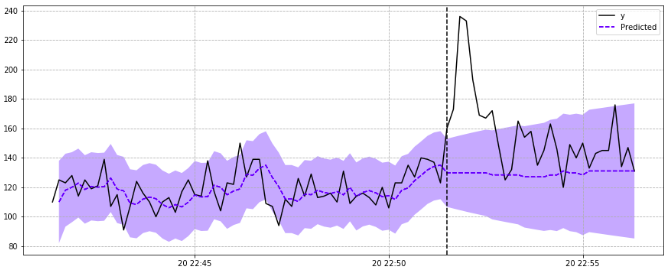The idea is to used the predictions of the fitted model (depicted in blue) as a reference to what probably would had been observed with no intervention taking place.

Bayesian Structural Time Series models can be expressed by the following equations:

$y_t = Z^T_t\alpha_t + \beta X_t + G_t\epsilon_t$
$a_{t+1} = T_t\alpha_t + H_t\eta_t$
$\epsilon_t \sim \mathcal{N}(0, \sigma_t^2)$
$\eta_t \sim \mathcal{N}(0, Q_t)$

The $a$ is also referred as a "state" of the series and $y_t$ is a linear combination of the states plus a linear regression with the covariates $X$ (and the measurement noise $\epsilon$ that follows a zero-mean normal distribution).

By varying the matricex $Z$, $T$, $G$ and $H$ we can model several distinct behaviors for the time series (including the more well known such as ARMA or ARIMA).

In this package (the same is true for Google's R package), you can choose any time series model you want to fit your data (more about this later below). If no model is used as input, a local level is built by default, which means $y_t$ is expressed as:

$y_t = \mu_t + \gamma_t + \beta X_t + \epsilon_t$
$\mu_{t+1} = \mu_t + \eta_{\mu, t}$

Any given point in time is modeled first by a random walk component $\mu_t$, also known as the "local level" component, which increases as the other arguments doesn't add much signal into explaining the data (as it models just randomness, there's no information being added with this component except the expectation for greater uncertainty).

We then have $\gamma_t$ which models seasonal components. This package uses the same model as the frequency one described in statsmodels:

\begin{split}\gamma_t & = \sum_{j=1}^h \gamma_{j, t} \\ \gamma_{j, t+1} & = \gamma_{j, t}\cos(\lambda_j) + \gamma^{*}_{j, t}\sin(\lambda_j) + \omega_{j,t} \\ \gamma^{*}_{j, t+1} & = -\gamma^{(1)}_{j, t}\sin(\lambda_j) + \gamma^{*}_{j, t}\cos(\lambda_j) + \omega^{*}_{j, t}, \\ \omega^{*}_{j, t}, \omega_{j, t} & \sim N(0, \sigma_{\omega^2}) \\ \lambda_j & = \frac{2 \pi j}{s}\end{split}

Finally we have the component $\beta X_t$ which is a linear regression of covariates that further helps to explain observed data. The better this component works into the prediction task, the lower the local level component should be.

The parameter $\epsilon_t$ models noise related to measuring $y_t$ and it follows a normal distribution with zero mean and $\sigma_{\epsilon}$ standard deviation.

## 2. How to Use¶

First, let's import and prepare the notebook for the code development:

In :
from IPython.core.display import HTML

def css_styling():
return HTML(styles)

css_styling()

Out:
In :
%matplotlib inline

import sys
import os

sys.path.append(os.path.abspath('../'))

import numpy as np
import pandas as pd
from IPython.core.pylabtools import figsize
figsize(14, 6)
import statsmodels as sm
from statsmodels.tsa.statespace.structural import UnobservedComponents
from statsmodels.tsa.arima_process import ArmaProcess
from matplotlib import pyplot as plt
from causalimpact import CausalImpact
import warnings

warnings.filterwarnings('ignore')


### 2.1 Generating Sample Data¶

In :
# This is an example presented in Google's R code.
np.random.seed(12345)
ar = np.r_[1, 0.9]
ma = np.array()
arma_process = ArmaProcess(ar, ma)

x0 = 100 + arma_process.generate_sample(nsample=100)
x1 = 90 + arma_process.generate_sample(nsample=100)
y = 1.2 * x0 + 0.9 * x1 + np.random.normal(size=100)
y[70:] += 5
data = pd.DataFrame({'x0': x0, 'x1': x1, 'y': y}, columns=['y', 'x0', 'x1'])

data.plot()
plt.axvline(69, linestyle='--', color='k')
plt.legend();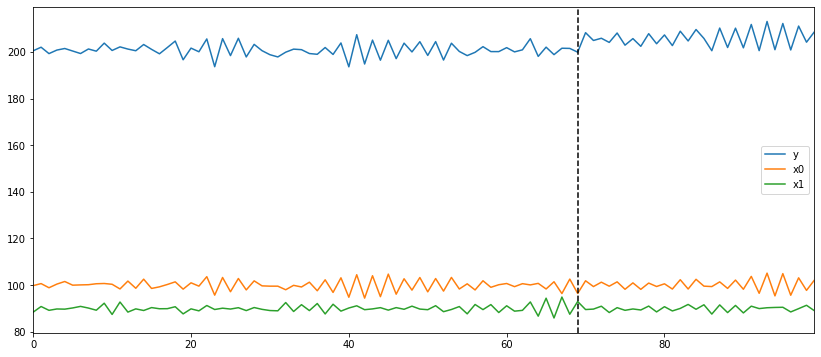### 2.1 Using Default Model¶

In :
pre_period = [0, 69]
post_period = [70, 99]

ci = CausalImpact(data, pre_period, post_period)

In :
ci.plot(figsize=(12, 6))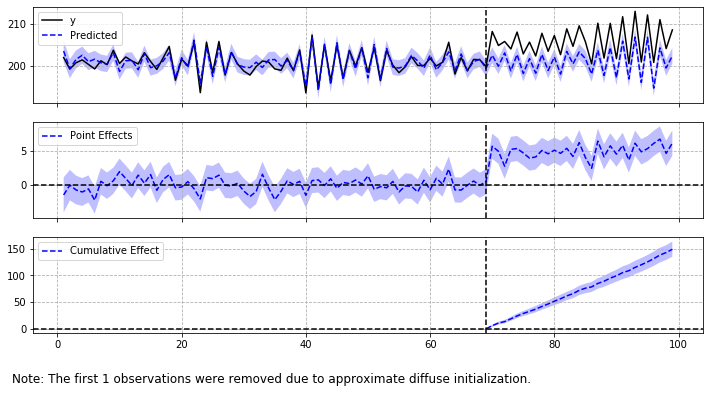When plotting results, three graphics are printed by default:

• the "origial" series versus its predicted one
• the "points effects" (which is the difference between original series and predicted)
• finally the "cumulative" effect which is basically the summation of the point effects accumulated over time.

You can choose which pannels to print; here's an example of just the original series and its point-wise effect printed out:

In :
ci.plot(panels=['original', 'pointwise'], figsize=(15, 12))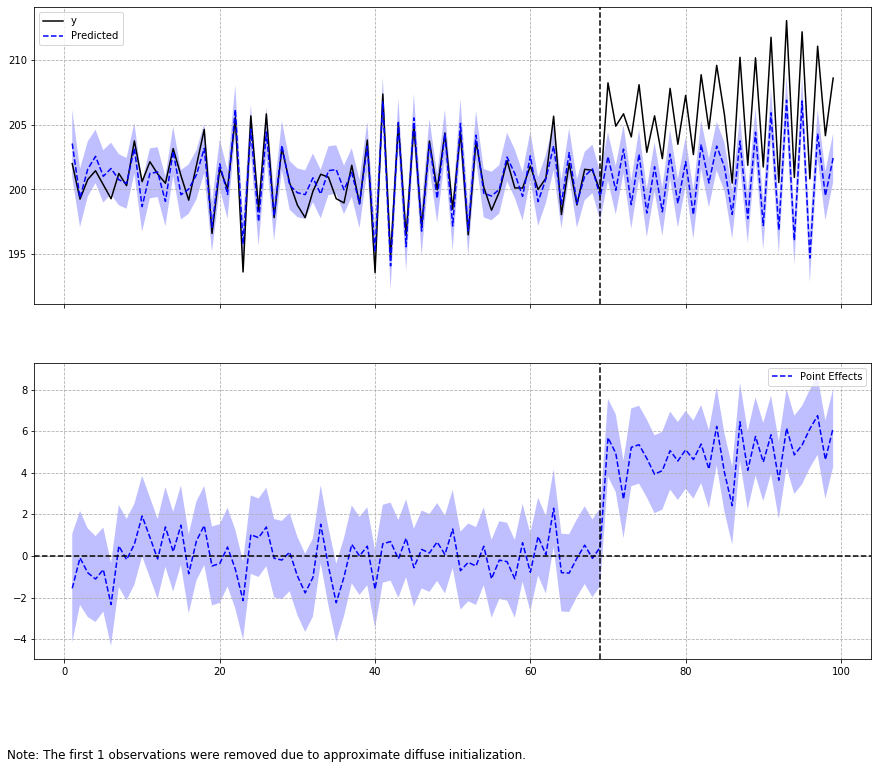For also viewing general results and numbers, you can invoke the summary method with either default input or "report" which prints a more detailed explanation of the observed effects:

In :
print(ci.summary())

Posterior Inference {Causal Impact}
Average            Cumulative
Actual                    206.0              6179.6
Prediction (s.d.)         201.0 (0.2)        6031.0 (7.3)
95% CI                    [200.6, 201.5]     [6016.5, 6045.0]

Absolute effect (s.d.)    5.0 (0.2)          148.6 (7.3)
95% CI                    [4.5, 5.4]         [134.6, 163.0]

Relative effect (s.d.)    2.5% (0.1%)        2.5% (0.1%)
95% CI                    [2.2%, 2.7%]       [2.2%, 2.7%]

Posterior tail-area probability p: 0.0
Posterior prob. of a causal effect: 100.00%

For more details run the command: print(impact.summary('report'))


We can see here for instance that the absolute effect observed is of 5.0 whose predictions varies from 4.5 up to 5.4 with 95% confidence interval.

A very important number to also consider when observing these results is the p-value or the probability of having a causal effect indeed (and not just noise). Remember to use this value in your analysis before taking conclusions!

If you want a more detailed summary, run the following:

In :
print(ci.summary(output='report'))

Analysis report {CausalImpact}

During the post-intervention period, the response variable had
an average value of approx. 206.0. By contrast, in the absence of an
intervention, we would have expected an average response of 201.0.
The 95% interval of this counterfactual prediction is [200.6, 201.5].
Subtracting this prediction from the observed response yields
an estimate of the causal effect the intervention had on the
response variable. This effect is 5.0 with a 95% interval of
[4.5, 5.4]. For a discussion of the significance of this effect,
see below.

Summing up the individual data points during the post-intervention
period (which can only sometimes be meaningfully interpreted), the
response variable had an overall value of 6179.6.
By contrast, had the intervention not taken place, we would have expected
a sum of 6031.0. The 95% interval of this prediction is [6016.5, 6045.0].

The above results are given in terms of absolute numbers. In relative
terms, the response variable showed an increase of +2.5%. The 95%
interval of this percentage is [2.2%, 2.7%].

This means that the positive effect observed during the intervention
period is statistically significant and unlikely to be due to random
fluctuations. It should be noted, however, that the question of whether
this increase also bears substantive significance can only be answered
by comparing the absolute effect (5.0) to the original goal
of the underlying intervention.

The probability of obtaining this effect by chance is very small
(Bayesian one-sided tail-area probability p = 0.0).
This means the causal effect can be considered statistically
significant.


The ci object also carries all the results of the fitting process, you can access all these values if you want to further analyze results:

In :
ci.inferences.head()

Out:
post_cum_y preds post_preds post_preds_lower post_preds_upper preds_lower preds_upper post_cum_pred post_cum_pred_lower post_cum_pred_upper point_effects point_effects_lower point_effects_upper post_cum_effects post_cum_effects_lower post_cum_effects_upper
0 NaN 199.256404 NaN NaN NaN -5316.556223 5715.069030 NaN NaN NaN 1.216337 -5514.596290 5517.028963 NaN NaN NaN
1 NaN 203.542200 NaN NaN NaN 200.933131 206.151269 NaN NaN NaN -1.552851 -4.161920 1.056218 NaN NaN NaN
2 NaN 199.344827 NaN NaN NaN 197.085073 201.604581 NaN NaN NaN -0.090130 -2.349884 2.169623 NaN NaN NaN
3 NaN 201.573546 NaN NaN NaN 199.442809 203.704282 NaN NaN NaN -0.804714 -2.935450 1.326022 NaN NaN NaN
4 NaN 202.552743 NaN NaN NaN 200.489453 204.616032 NaN NaN NaN -1.108351 -3.171640 0.954939 NaN NaN NaN

You can also retrieve information related to the trained model (such as what were the fitted parameters and so on):

In :
ci.trained_model.params

Out:
sigma2.irregular    0.111837
sigma2.level        0.000069
beta.x0             0.945247
beta.x1             0.502555
dtype: float64

It's also possible to choose a prior value to the standard deviation of the level parameter (or any parameter you'd like to set boundaries to). Here's an example:

In :
ci = CausalImpact(data, pre_period, post_period, prior_level_sd=0.1)

In :
ci.plot(figsize=(15, 12))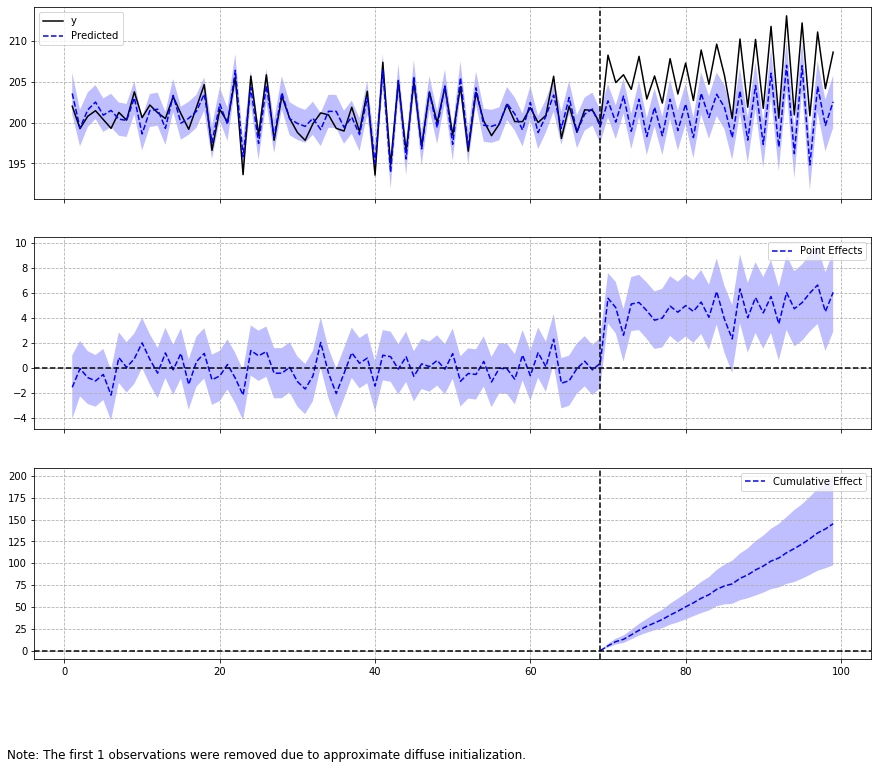Default value is $0.01$ which represents data well behaved with small variance and well explained by the covariates. If it turns out to not to be the case for a given input data, it's possible to change the value on the prior of the local level standard deviation to reflect this pattern in data. For the value $0.1$, we are basically saying that there's a stronger component of a random walk in data that cannot be explained by the covariates themselves.

It's also possible to model season components in data. First, let's use a season simulator available in statsmodels package:

In :
def simulate_seasonal_term(periodicity, total_cycles, noise_std=1.,
harmonics=None):
duration = periodicity * total_cycles
assert duration == int(duration)
duration = int(duration)
harmonics = harmonics if harmonics else int(np.floor(periodicity / 2))

lambda_p = 2 * np.pi / float(periodicity)

gamma_jt = noise_std * np.random.randn((harmonics))
gamma_star_jt = noise_std * np.random.randn((harmonics))

total_timesteps = 100 * duration # Pad for burn in
series = np.zeros(total_timesteps)
for t in range(total_timesteps):
gamma_jtp1 = np.zeros_like(gamma_jt)
gamma_star_jtp1 = np.zeros_like(gamma_star_jt)
for j in range(1, harmonics + 1):
cos_j = np.cos(lambda_p * j)
sin_j = np.sin(lambda_p * j)
gamma_jtp1[j - 1] = (gamma_jt[j - 1] * cos_j
+ gamma_star_jt[j - 1] * sin_j
+ noise_std * np.random.randn())
gamma_star_jtp1[j - 1] = (- gamma_jt[j - 1] * sin_j
+ gamma_star_jt[j - 1] * cos_j
+ noise_std * np.random.randn())
series[t] = np.sum(gamma_jtp1)
gamma_jt = gamma_jtp1
gamma_star_jt = gamma_star_jtp1
wanted_series = series[-duration:] # Discard burn in

return wanted_series

In :
duration = 100
periodicities = [7, 30]
num_harmonics = [2, 5]
std = np.array([0.1, 0.1])
# np.random.seed(8678309)

terms = []
for ix, _ in enumerate(periodicities):
s = simulate_seasonal_term(
periodicities[ix],
duration / periodicities[ix],
harmonics=num_harmonics[ix],
noise_std=std[ix])
terms.append(s)
terms.append(np.ones_like(terms) * 10.)
seasons = pd.Series(np.sum(terms, axis=0))

In :
seasons.plot(label='season periods (7) + (30)', legend=True);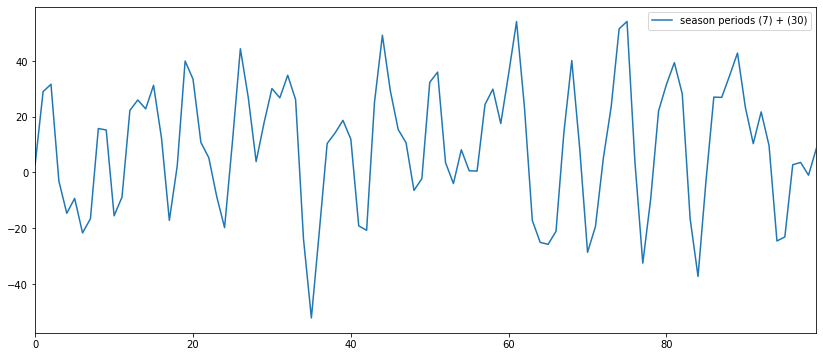In :
season_data = data.copy()
season_data['y'] += seasons

In :
ci = CausalImpact(season_data, pre_period, post_period,
nseasons=[{'period': 7, 'harmonics': 2}, {'period': 30, 'harmonics': 5}])

In :
print(ci.summary())

Posterior Inference {Causal Impact}
Average            Cumulative
Actual                    215.2              6455.4
Prediction (s.d.)         211.0 (0.8)        6329.4 (24.5)
95% CI                    [209.4, 212.6]     [6282.4, 6378.5]

Absolute effect (s.d.)    4.2 (0.8)          125.9 (24.5)
95% CI                    [2.6, 5.8]         [76.9, 172.9]

Relative effect (s.d.)    2.0% (0.4%)        2.0% (0.4%)
95% CI                    [1.2%, 2.7%]       [1.2%, 2.7%]

Posterior tail-area probability p: 0.0
Posterior prob. of a causal effect: 100.00%

For more details run the command: print(impact.summary('report'))


### 2.2 Working with Dates Index¶

The model should work with dates as well. Let's see what happens when our input data have a date as index type:

In :
dated_data = data.set_index(pd.date_range(start='20180101', periods=len(data)))

pre_period = ['20180101', '20180311']
post_period = ['20180312', '20180410']

figsize = (20, 6)
ci = CausalImpact(dated_data, pre_period, post_period)

In :
ci.plot(figsize=(15, 14))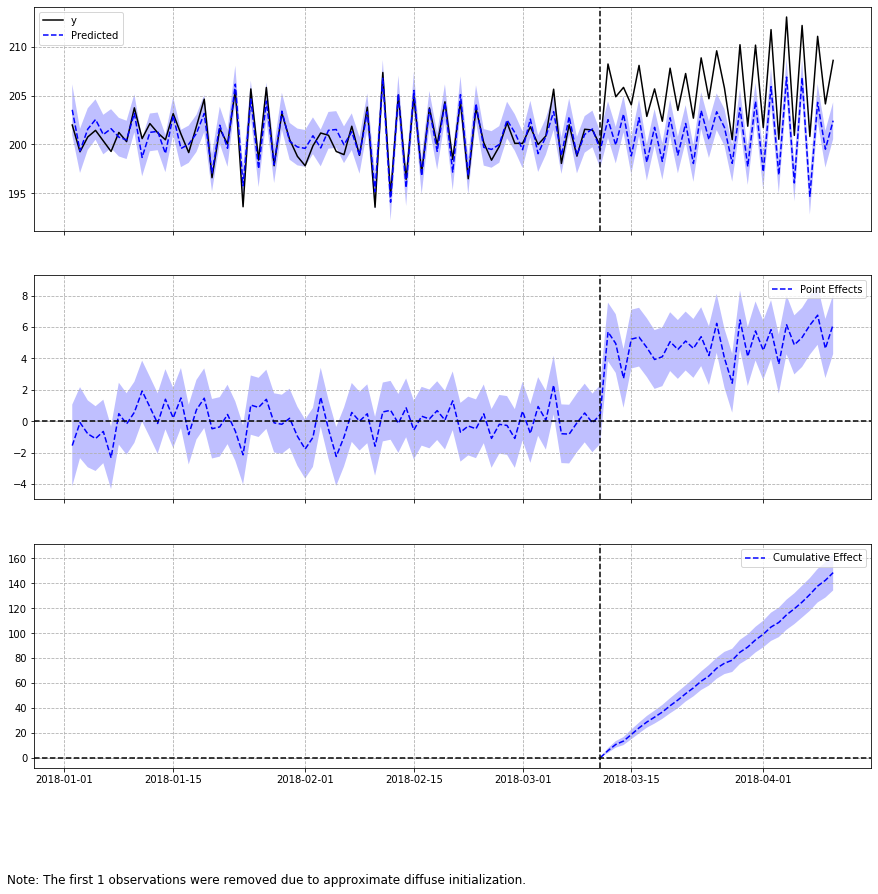In :
print(ci.summary())

Posterior Inference {Causal Impact}
Average            Cumulative
Actual                    206.0              6179.6
Prediction (s.d.)         201.0 (0.2)        6031.0 (6.8)
95% CI                    [200.6, 201.5]     [6018.0, 6044.8]

Absolute effect (s.d.)    5.0 (0.2)          148.6 (6.8)
95% CI                    [4.5, 5.4]         [134.8, 161.6]

Relative effect (s.d.)    2.5% (0.1%)        2.5% (0.1%)
95% CI                    [2.2%, 2.7%]       [2.2%, 2.7%]

Posterior tail-area probability p: 0.0
Posterior prob. of a causal effect: 100.00%

For more details run the command: print(impact.summary('report'))


### 2.3 Customized Model¶

Just as in Google R package, here you can also choose a customized model to be trained in the pre-intervention period. It's important to note that if you want to have the data standardized, then your customized model must be built with data already standardized and the input data must be the original denormalized data, like so:

In :
from causalimpact.misc import standardize

normed_pre_data, _ = standardize(data.iloc[:70])

model = UnobservedComponents(
endog=normed_pre_data.iloc[:70, 0],
level='llevel',
exog=normed_pre_data.iloc[:70, 1:]
)

pre_period = [0, 69]
post_period = [70, 99]

ci = CausalImpact(data, pre_period, post_period, model=model)

In :
ci.plot(figsize=(15, 12))